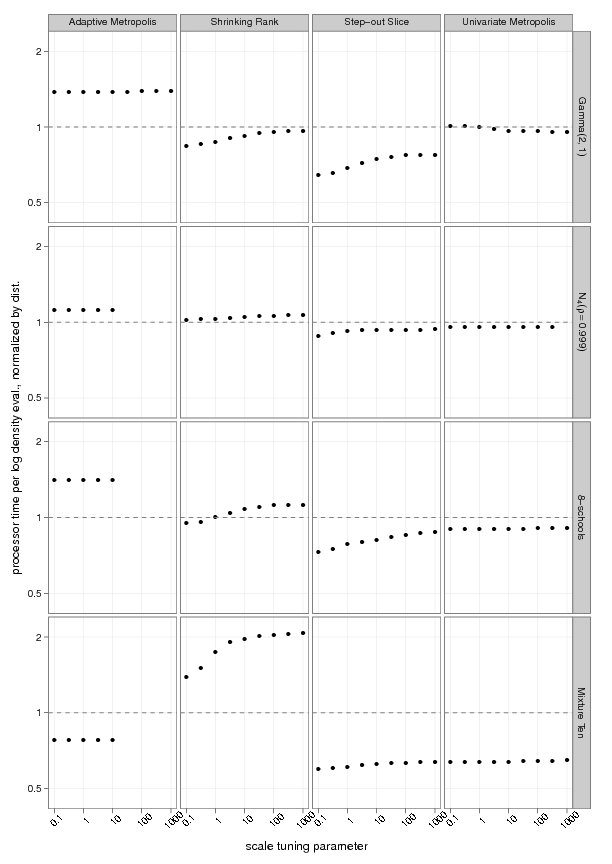Want to share your content on R-bloggers? click here if you have a blog, or here if you don't.A new posting on arXiv by Madeleine Thompson on a graphical tool for assessing performance. She has developed a software called SamplerCompare, implemented in R and C. The graphical evaluation plots “log density evaluations per iteration times autocorrelation time against a tuning parameter in a grid of plots where rows represent distributions and columns represent methods”. The autocorrelation time is evaluated in the same way as coda, which is the central package used in the convergence assessment chapter of Introducing Monte Carlo Methods with R because of its array of partial (if imperfect) indicators. Note that there is an approximation factor in the evaluation of the autocorrelation time because the MCMC output is represented as an AR(p) series, with a possible divergence artifact in the corresponding confidence interval if the AR(p) process is found to be non-stationary. When the simulation method (corresponding to columns in the above graphs) allows for an optimal value of its (cyber-)parameters, the performances exhibit a clear parabolic pattern (right graph), but this is not always the case (left graph). Graphical tools are always to be preferred to tables (a point Andrew would not rebuke!), However I do not see the point in simultaneously graphing the performances of different MCMC algorithms for different targets. This “wasted” dimension could instead be used for increasing to at least three the number of cyber-parameters evaluated by the method.

Filed under: Books, R, Statistics Tagged: adaptive MCMC methods, autocorrelation, coda, Introducing Monte Carlo Methods with R, MCMC algorithms, R, simulation